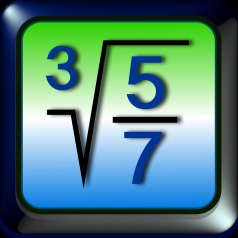iMathics

# Messages

short message

division by zero

decimal overflow

result is undefined

result is imaginary

missing exponent

missing numerator

missing denominator

missing base value

missing input values

missing right paranthesis

missing left paranthesis

root extracting error

exponentiation error

exponentiation error

division error

logarithm error

logarithm error

logarithm error

missing argument

argument invalid

argument invalid

argument invalid

argument invalid

argument invalid

long message

Result invalid. Reason: division by zero.

The result is greater then the greatest representable number

Reason: 0th power of 0 is not defined

extracting the root from a negative number

Explanation: If you extract the root of a negative number, the result is undefined

base value is missing: please insert

Explanation: The base value of a logarithm, a root or an exponentiation is missing.

Several input values are missing: please insert

Explanation: Several input values e.g. numerators, denominators, exponents are missing.

more left then right parantheses; please correct

more right then leftt parantheses; please correct

root exponent is zero

Explanation: A zero root exponent implies a division with zero, and the result is undefined.

zero raised to a negative power

Explanation: Zero raised to a negative power implies a division with zero, and the result is undefined.

a negative number raised to an irrational power

Explanation: An irrational power of a negative number is undefined.

zero divided by zero is undefined.

the argument of logarithm must be positive.

Explanation: The logarithm function is defined only for positive arguments.

the base of logarithm must be positive.

Explanation: The logarithm function is defined only for positive base values.

both the base and the argument of logarithm must be positive

Explanation: The logarithm function is defined only for positive arguments and positive base values.

Explanation: The argument of a function (e. g. sin, cos, tan) is missing

argument of arcsin or arccos must be in the interval [-1,+1]

Explanation: The argument of arcsin or arccos must be greater then or equal to -1 and less then or equal to +1.

argument of tan must not be 90° or an odd multiply of it

argument of tan must not be π/2 or an odd multiply of it

argument of arcosh must be greater than or equal to 1

argument of artanh must be less then 1 and greater than -1